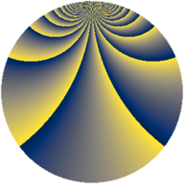# Properties

 Label 1183.2.btLevel $1183$ Weight $2$ Character orbit 1183.bt Rep. character $\chi_{1183}(36,\cdot)$ Character field $\Q(\zeta_{78})$ Dimension $2160$ Sturm bound $242$

# Related objects

## Defining parameters

 Level: $$N$$ $$=$$ $$1183 = 7 \cdot 13^{2}$$ Weight: $$k$$ $$=$$ $$2$$ Character orbit: $$[\chi]$$ $$=$$ 1183.bt (of order $$78$$ and degree $$24$$) Character conductor: $$\operatorname{cond}(\chi)$$ $$=$$ $$169$$ Character field: $$\Q(\zeta_{78})$$ Sturm bound: $$242$$

## Dimensions

The following table gives the dimensions of various subspaces of $$M_{2}(1183, [\chi])$$.

Total New Old
Modular forms 2976 2160 816
Cusp forms 2880 2160 720
Eisenstein series 96 0 96

## Trace form

 $$2160q - 88q^{4} + 18q^{6} + 88q^{9} + O(q^{10})$$ $$2160q - 88q^{4} + 18q^{6} + 88q^{9} - 4q^{10} - 6q^{11} - 12q^{12} - 4q^{13} + 8q^{14} + 46q^{15} + 84q^{16} + 4q^{17} - 156q^{18} + 12q^{20} + 38q^{22} - 142q^{23} - 168q^{24} + 184q^{25} + 42q^{26} - 12q^{27} - 10q^{29} - 364q^{30} + 104q^{31} + 120q^{32} + 30q^{33} + 130q^{34} - 8q^{35} - 74q^{36} + 42q^{37} - 4q^{38} - 48q^{39} + 112q^{40} - 30q^{41} - 4q^{42} + 16q^{43} - 130q^{45} - 12q^{46} - 286q^{47} + 64q^{48} - 90q^{49} + 18q^{50} + 78q^{51} - 132q^{52} + 22q^{53} - 12q^{54} - 166q^{55} + 12q^{56} + 78q^{57} + 64q^{58} - 18q^{59} - 208q^{60} - 14q^{61} - 114q^{62} - 12q^{63} + 164q^{64} - 60q^{65} - 108q^{66} + 76q^{67} - 4q^{68} + 12q^{69} + 24q^{71} + 278q^{72} + 18q^{74} - 30q^{75} - 398q^{76} - 8q^{77} - 250q^{78} + 76q^{79} + 72q^{80} + 66q^{81} - 246q^{82} - 520q^{83} - 18q^{84} + 230q^{85} + 202q^{87} - 458q^{88} + 12q^{89} - 120q^{90} - 14q^{91} + 16q^{92} - 112q^{93} + 60q^{94} - 72q^{95} - 338q^{96} - 6q^{97} + O(q^{100})$$

## Decomposition of $$S_{2}^{\mathrm{new}}(1183, [\chi])$$ into newform subspaces

The newforms in this space have not yet been added to the LMFDB.

## Decomposition of $$S_{2}^{\mathrm{old}}(1183, [\chi])$$ into lower level spaces

$$S_{2}^{\mathrm{old}}(1183, [\chi]) \cong$$ $$S_{2}^{\mathrm{new}}(169, [\chi])$$$$^{\oplus 2}$$